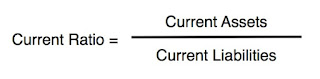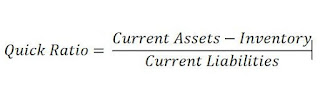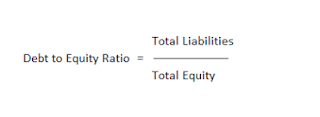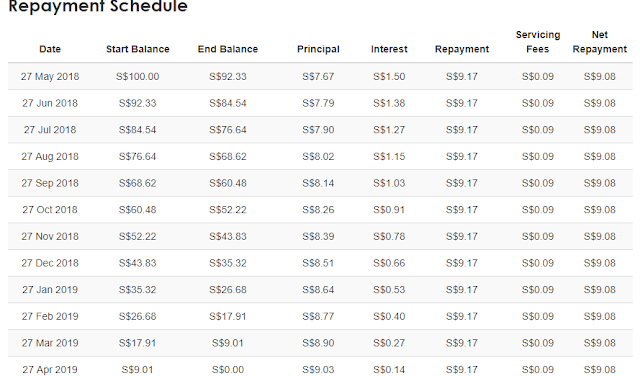# (Post 86/Year 1 week 23)Investment project updates(Peer to peer lending):Moolahsense(My thirteen and last campaign)Moolahsense(My thirteen and last campaign with Moolahsense)

Note*the company name will not be revealed just like the previous post as my money are still inside moolahsense, haha(this is not a sponsored post by the way)

Issuer summary
Date of listing:April 2018
Amount:S\$100,000.00
Tenor: 12 months
Note type: Equal installment

Quoting from the moolahsense website

• Equal installment:A promissory note by which an installment of the principal subscription amoung and/or interest accrued and due up to the date of payment shall be payable on such dates as set out in a repayment schedule specified in the promissory note, and on the maturity date, the principal subscription amount would have been paid in full

Repayment frequency: Monthly

• Repayment frequency: Will be paid monthly

Target interest rate:18%P.A

Quoting from Moolahsense website

• Target interest rate: The maximum rate the issuer is willing to provide the interest on the principal subscription amount which the issuer wished to obtain, as notified in writing by the issue to Moolahsese

Purpose: Working capital

Quote from valuepenguin:https://www.valuepenguin.sg/what-is-working-capital

• Working capital: It is a concept to describe a business ability to cover its short-term operating costs
• E.g For this campaign, I have funded \$100 into it
• As the target interest rate is 18% PA, this means that at the end of the tenor, which is 6 month I will expect to receive \$118
• 100%=\$100
• 18%(Interest for 12 month)=\$18.00
• 118%(The Principal + the interest)=\$100+ \$18.00=\$118.00
• Since the tenor is 12 months, each month I will receive \$9.83(inclusive of interest) in payment/equal installment
• 1month=\$118.00/12=\$9.83

Summary company profile

• The issuer is a company incorporated in Singapore in 2014
• The Issuer is in the food and beverages industry and has around 10 employees.
• The Issuer specializes in the retail sale of snack food products (including chips, cookies, chocolates and candies) sourced primarily from Japan.

Detail of purpose

• The Issuer seeks working capital for the performance of various contracts which have been awarded to the Issuer.

Financial statement

Will only reveal a part of the financial statement due to confidentiality. As I have mentioned a few weeks ago in my post, that I only mainly use this three ratio: current ratio, quick ratio and debt to equity ratio to decide in investing in a campaign, hence I will be showing its ratio below

The ratio is as follow(left side of the column is for the year 2017/right side of the column is for the year 2016)Year 2017/Year 2016
(Current ratio 2017:8.53),(Current ratio 2016:10.49)• A simple ratio of current asset divide by current liabilities
• Current liabilities are debt that needs to clear in the short term(in a year)
• If a company has a current ratio less then 1.0, do not invest in it
• If a company has a current ratio more then 2.0, May consider investing in it
• The higher the current ratio, the better
• For the current ratio of 2016:8.53, it is higher than the recommended range(>2.0)
• For the current ratio of 2017:10.49, it is higher than the recommended range(>2.0)
(Quick ratio 2016:6.52),(Quick ratio 2015:9.54)• The quick ratio is almost similar to current ratio except that it is assumed that the company does not sell its inventories(e.g Toyota inventory is its car) or stock, it is still able to fulfill its debt
• If the company has a quick ratio of 0.75 and below, do not invest in it
• If the company has a quick ratio of 1.25 and above, May consider investing in it
• The higher the quick ratio the better
• For the quick ratio of 2016:6.52, it is higher than the recommended range(>1.25)
• For the quick ratio of 2017:9.54, it is higher than the recommended range(>1.25)

• (Total liabilities/equity 2016:0.3),(Total liabilities/equity 2015:0.41)• The debt ratio is calculated by total liabilities divided by the equity
• If the company has a debt ratio of 1.5 and above, do not invest in it
• The company should essentially have a debt ratio of 1.0, if the debt ratio is below 0.75, do consider investing in it
• The lower the debt to equity ratio the better
• For the Total liabilities/equity of 2016:0.3 it is within the recommended range(<0.75)
• For the Total liabilities/equity of 2017:0.41, it is within the recommended range(<0.75)

Why did I invest in this loan?
• All current ratio,quick ratio and total liabilities/equity ratio meet the requirement,hence invested in it
Repayment schedule?link on how too read the effective interest rate for moolahsense:http://letscrowdsmarter.com/understanding-interest-rates/
Monthly interest rate:interest/start balance=\$1.50/\$100=1.5%(Percentage conversion must *100)
Effective interest rate:1.5%*12=18% same as the target interest rate at 18%(see the top of the post))
From the above picture, you can see that the Net repayment is at \$9.08 instead of the \$9.83 that I have mentioned above at the working capital, hence I will do a calculation here again
1-month repayment:\$9.08
12-month repayment:\$9.08*12=\$108.96
\$100(the amount I put in this campaign=100%
\$108.96=(100/100)*108.96=108.96
108.96%-100%=8.96%
The target interest rate as you can see in my above post is at around 18%P.A, after taking into account of the tenor rate being 12 months, which is 18 % and comparing it to 8.96% its almost 10% difference(i shall call this net interest rate instead)in this campaign. To conclude, this means that if you invest in a (18%P. A) high-interest rate campaign in moolahsense which has a tenor rate for 12 months, you will get only about 8.96%
The reason for quitting moolahsense is because I prefer investing in stock and also some of the delinquent payment via moolahsense, that’s why this is the last campaign in moolahsense, who knows i will return in the future?
Do look out for future update on this blog!

Leave a Reply

Your Name (required)

Your Email (required)

Your Message